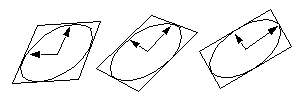### 2.5.6 Ellipses and Elliptical Arcs in CLIM

An ellipse is an area that is the outline and interior of an ellipse. Circles are special cases of ellipses.

An elliptical arc is a path consisting of all or a portion of the outline of an ellipse. Circular arcs are special cases of elliptical arcs.

An ellipse is specified in a manner that is easy to transform, and treats all ellipses on an equal basis. An ellipse is specified by its center point and two vectors that describe a bounding parallelogram of the ellipse. The bounding parallelogram is made by adding and subtracting the vectors from the center point in the following manner:

###### Bounding Parallelogram of an Ellipse

x coordinate

y coordinate

Center of Ellipse

x c

y c

Vectors

dx 1

dx 2

dy 1

dy 2

Corners of Parallelogram

x c + dx 1 + dx 2

x c + dx 1 - dx 2

x c - dx 1 - dx 2

x c - dx 1 + dx 2

y c + dy 1 + dy 2

y c + dy 1 - dy 2

y c - dy 1 - dy 2

y c - dy 1 + dy 2

The special case of an ellipse with its axes aligned with the coordinate axes can be obtained by setting dx 2 and dy 1 to 0, or setting dx 1 and dy 2 to 0.

Note that several different parallelograms specify the same ellipse, as shown here:###### Figure 12. Ellipses Specified by Parallelograms

One parallelogram is bound to be a rectangle--the vectors will be perpendicular and correspond to the semi-axes of the ellipse.

The following classes and functions are used to represent and operate on ellipses and elliptical arcs.

`ellipse [Protocol Class]	`

Summary: The protocol class that corresponds to a mathematical ellipse. This is a subclass of area . If you want to create a new class that behaves like an ellipse, it should be a subclass of ellipse . Subclasses of ellipse must obey the ellipse protocol.

`ellipsep [Function]	`

Arguments: object

Summary: Returns t if object is an ellipse; otherwise, it returns nil .

standard-ellipse

Summary: An instantiable class that implements an ellipse. This is a subclass of ellipse . This is the class that make-ellipse and make-ellipse* instantiate. Members of this class are immutable.

`elliptical-arc [Protocol Class]	`

Summary: The protocol class that corresponds to a mathematical elliptical arc. This is a subclass of path . If you want to create a new class that behaves like an elliptical arc, it should be a subclass of elliptical-arc . Subclasses of elliptical-arc must obey the elliptical arc protocol.

`elliptical-arc-p [Function]	`

Arguments: object

Summary: Returns t if object is an elliptical arc; otherwise, it returns nil .

standard-elliptical-arc

Summary: An instantiable class that implements an elliptical arc. This is a subclass of elliptical-arc . This is the class that make-elliptical-arc and make-elliptical-arc* instantiate. Members of this class are immutable.

#### 2.5.6.2 Accessors for CLIM Elliptical Objects

Common Lisp Interface Manager 2.0 User's Guide - 7 Aug 2017